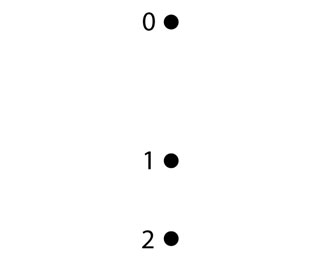# Problem: The following figure shows the first three points of a motion diagram.Is the object’s average speed between points 1 and 2 greater than, less than, or equal to its average speed between points 0 and 1?Find the average acceleration vector at point 1. Draw the completed motion diagram, showing the velocity vectors and acceleration vector (v0 is velocity between points 0 and 1 and v1 is velocity between points 1 and 2).

⚠️Our tutors found the solution shown to be helpful for the problem you're searching for. We don't have the exact solution yet.

###### Problem Details

The following figure shows the first three points of a motion diagram.Is the object’s average speed between points 1 and 2 greater than, less than, or equal to its average speed between points 0 and 1?

Find the average acceleration vector at point 1. Draw the completed motion diagram, showing the velocity vectors and acceleration vector (v0 is velocity between points 0 and 1 and v1 is velocity between points 1 and 2).